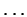Next: 1.2.4 Java Statements Up: 1.2 Java Mechanics Previous: 1.2.2 Java Expressions

## 1.2.3 Precedence of Operations

Since Java uses conventional infix notation for expressions it relies on the notion of precedence to determine how expressions like

12 * 5 + 10
should be interpreted. The Java operations given in the preceding subsection are divided into the following precedence groups:
 prefix operators - ! multiplicative star / % additive + - relational < > >= <= equality == != logical and && logical or || conditional ?:
from highest to lowest. A higher precedence operator has greater ``binding power''. For example, the expression
72. - 32. * 1.8
is equivalent to
72. - (32. * 1.8)
because star has higher precedence than infix -.

Finger Exercise: In the DrJava Interactions window: available, try evaluating the following expressions:

```72. - 32. * 1.8
(72. - 32.) * 1.8
```

All of infix operators listed above are left-associative: when infix operators of equal precedence are chained together, the leftmost operator has precedence. For example,

72. - 30. - 12.
is equivalent to
(72. - 30.) - 12.
Parentheses can be used to override the built-in precedence and associativity of operators. Hence,
(72. - 32.) * 1.8
equals 40*1.8. Similarly,
72. - (30. - 12.)
equals
72. - 18.
It is a good idea to use parentheses if you have any doubts about the precedence relationship between consecutive operators. The judicious use of parentheses makes complex expressions easier to read.

Finger Exercise: In the DrJava Interactions window, try evaluating the following expressions:

```72. - 30. - 12.
72. - (30. - 12.)
```Next: 1.2.4 Java Statements Up: 1.2 Java Mechanics Previous: 1.2.2 Java Expressions
Corky Cartwright
2000-01-07